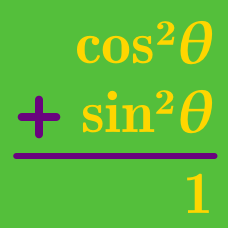Geometry

# Basic Proving Identities using Pythagorean Theorem

Which of the following is equal to $$1-\cos^2\theta?$$

Which of the following is equal to $$1-\sin^2\theta?$$

Which of the following is equal to $$(1-\cos\theta)(1+\cos\theta)?$$

Which of the following is equal to $$(\sin\theta-1)(\sin\theta+1)?$$

Which of the following is equal to $$\dfrac{\sec^2\theta}{\tan^2\theta+1}?$$

×